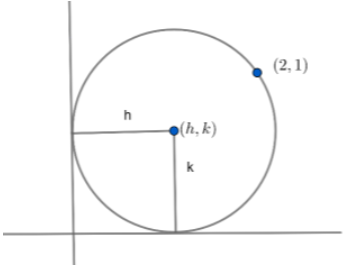Courses
Courses for Kids
Free study material
Free LIVE classes
MoreLIVE
Join Vedantu’s FREE Mastercalss

# Find the equations of the circle touching both axes and passing through the point$\left( 2,1 \right)$.Verified
365.1k+ views
Hint: It is given that the circle touches both the $x$ and $y$ axis. Using this information given in the question, we can draw the figure and we can find the relation between coordinates of the centre of the circle and the radius of the circle.

It is given in the question that the circle is touching $x=0$i.e. $y$ - axis and $y=0$ i.e. $x$ - axis and also passing through the point $\left( 2,1 \right)$.

Let us assume a circle having it’s centre at the coordinate $\left( h,k \right)$ and it’s radius equal to $r$.
The general equation of this circle is given by ${{(x-h)}^{2}}+{{(y-k)}^{2}}={{r}^{2}}$.
Since the circle is touching $x=0$ and $y=0$ and also passing through $\left( 2,1 \right)$, Using this data, we can now plot the circle.From the figure drawn above, we can find out that radius of the circle $r=h=k$.
$\Rightarrow k=h$ and $r=h$
So, we have a circle with centre $\left( h,h \right)$ and radius $=h$
So, from this information, equation of the circle
${{\left( x-h \right)}^{2}}+{{\left( y-h \right)}^{2}}={{h}^{2}}...........\left( i \right)$
$\Rightarrow {{x}^{2}}+{{h}^{2}}-2hx+{{y}^{2}}+{{h}^{2}}-2hy={{h}^{2}}$
Cancelling ${{h}^{2}}$ from left side and right side, we get 🡪$\Rightarrow {{x}^{2}}+{{y}^{2}}-2hx-2hy+{{h}^{2}}=0........\left( ii \right)$
Since $\left( 2,1 \right)$ lies on this circle, we can substitute $x=2$ and $y=1$ in above equation $\left( ii \right)$ ,
$\Rightarrow {{\left( 2 \right)}^{2}}+{{\left( 1 \right)}^{2}}-2\left( 2 \right)h-2\left( 1 \right)h+{{h}^{2}}=0$
$\Rightarrow {{h}^{2}}-4h-2h+1+4=0$ $\Rightarrow {{h}^{2}}-6h+5=0$
$\Rightarrow {{h}^{2}}-h-5h+5=0$
\begin{align} & \Rightarrow h\left( h-1 \right)-5\left( h-1 \right)=0 \\ & \Rightarrow \left( h-1 \right)\left( h-5 \right)=0 \\ & \Rightarrow h=5,h=1 \\ \end{align}
There are two possible values of $h$. So two possible circles corresponding to two possible values of
$h$. Substituting $h=5$ and $h=1$ in equation $\left( i \right)$, the equation of these two circles are
given by 🡪
${{\left( x-5 \right)}^{2}}+{{\left( y-5 \right)}^{2}}={{5}^{2}}$ and ${{\left( x-1 \right)}^{2}}+{{\left( y-1 \right)}^{2}}={{1}^{2}}$
$\Rightarrow {{\left( x-5 \right)}^{2}}+{{\left( y-5 \right)}^{2}}=25$ and ${{\left( x-1 \right)}^{2}}+{{\left( y-1 \right)}^{2}}=1$

Note: There is a possibility of committing a mistake while expanding ${{\left( x-h \right)}^{2}}$ in equation $\left( i \right)$ . There are chances that one may expand it as ${{x}^{2}}+{{h}^{2}}+2hx$ instead of ${{x}^{2}}+{{h}^{2}}-2hx$. This mistake will lead to the incorrect answer.

Last updated date: 27th Sep 2023
Total views: 365.1k
Views today: 10.65k